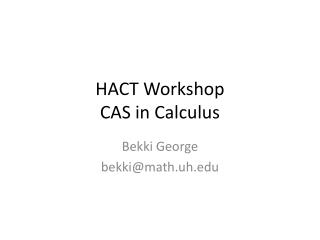DownloadDownload PresentationHACT Workshop CAS in Calculus

# HACT Workshop CAS in Calculus

Télécharger la présentation## HACT Workshop CAS in Calculus

- - - - - - - - - - - - - - - - - - - - - - - - - - - E N D - - - - - - - - - - - - - - - - - - - - - - - - - - -
##### Presentation Transcript

1. HACT WorkshopCAS in Calculus Bekki George bekki@math.uh.edu

2. AP Calculus Resource Site http://online.math.uh.edu/apcalculus/

3. Over 40 Online Quizzesand AP Practices Exams http://www.estudy.uh.edu/

4. Creating Your Own Online Tutorials Jing(free): http://www.techsmith.com/jing/

5. Jing – Student Projects • Students can create videos on current topics to share with the class

6. More Free Software • Sage • Geogebra • Winplot • GraphCalc • SpaceTime

7. Sage • http://sagemath.org • http://wiki.sagemath.org/interact/calculus • http://www.sagemath.org/library.html

8. What Can Sage Do? • Graphs f1=-x+2 f2=1/x^2 f=piecewise([[(-1,1),f1],[(1,3),f2]]) f.plot() • Calculate limits limit((x^2-25)/(x-5),x=5) derivatives f=sin(x^3)+x*cos(x) diff(f,x) // or diff(f,x,n) to find the nth derivative integrals integral(3*x^2,x) integral(x*sin(x^2),x,0,pi/2) • SAGE can verify laws the product rule function('f, g‘) diff(f(t)*g(t),t) and the quotient rule diff(f(t)/g(t), t) AND MUCH MORE!!!

9. Sage Quick Reference: Calculus http://wiki.sagemath.org/quickref

10. Geogebra • http://www.geogebra.org/

11. Create Dynamic Worksheets on Web http://www.math.uh.edu/~bekki/e_d_limits.html

12. http://www.math.uh.edu/~bekki/sectan.html

13. Text boxes from SecTan "Slope of the secant line through (" + a + "," + (f(a)) + ") and (" + (a + h) + "," + (f(a + h)) + ") :" ---------------------------------------- " \frac{f(" + a + "+h) - f(" + a + ")}{h} = " + m2 ----------------------------------------- "Slope of the tangent line at x=" + a + ":" ----------------------------------------- "\lim_{h \to 0} \frac{f(" + a + "+h) - f(" + a + ")}{h} = " + m Condition to show (under advanced): First two boxes: h>0 Last two boxes: h=0

14. Robert’s stuff www.dkjohnson.us/TriangleTilingBaseColors2.html

15. Winplot • http://math.exeter.edu/rparris/winplot.html

16. GraphCalc • http://www.graphcalc.com/

17. SpaceTime • http://www.spacetime.us/ • Workshop III -Online!November 13, 2010 • Jeff Morgan will present:Exploring Mathematics with SpaceTime 4.0